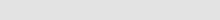Insilicase
deus ex computaGrams to addFinal volumeMaximum concentrationsStock solutionsOD to concOD to primer concProtein in DNA solnCentrifugation calculationsIsotope decayRadioactivity to molarityRadioactivity units conversionAny ideas?Contact me# Maths in Biology

Biomaths an Android application which contains the functions in thse pages can be found here on the Android Market Place / Google Play.

### Purpose of these pages

These pages allow you to perform simple calculations that are common enough for me to write this, but rare enough for everyone to forget how to do it.

### Making solutions

This page helps you calculate how much to add to make a solution of the right molarity
Mass calculation

This page helps you calculate how much of a solution you can make given an amount of the compound and the desired molarity
Volume calculation

This page helps you calculate the maximum concentration of a solution you can make for a given mass and volume
Molarity calculation

This page helps you calculate how much of a stock solution you need to use to make a working solution of a certain concentration
Stock solution calculation

### DNA concentrations

This page converts ODs in to nucleic acid concentrations for long sequences
OD > concentration

OD > primer concentration

This page calculates the amount of protein in a nucleic acid solution
Protein in a nucleic acid solution

### Centrifugation calculations

This page converts RPM to G force and G force to RPM for a given rotor radius
Centrifugation calculations

This page calculates the current activity of a radioactive compound given is inital activity, time since the activity date and the isotopes half life.